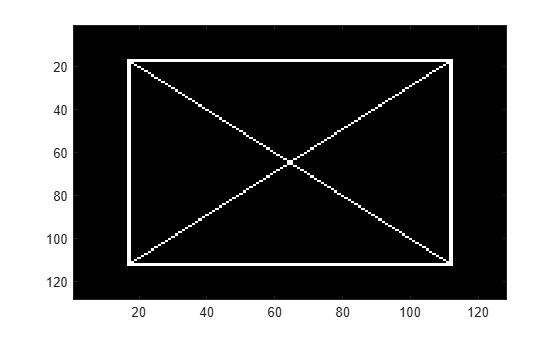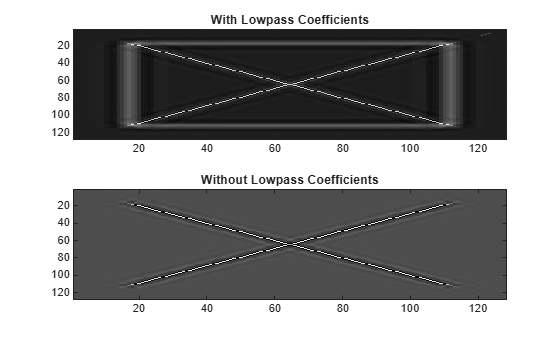# idualtree2

Kingsbury Q-shift 2-D inverse dual-tree complex wavelet transform

Since R2020a

## Syntax

``imrec = idualtree2(A,D)``
``imrec = idualtree2(___,Name,Value)``

## Description

````imrec = idualtree2(A,D)` returns the inverse 2-D complex dual-tree transform of the final-level approximation coefficients, `A`, and cell array of wavelet coefficients, `D`. `A` and `D` are outputs of `dualtree2`. For the reconstruction, `idualtree2` uses two sets of filters: Orthogonal Q-shift filter of length 10Near-symmetric biorthogonal filter pair with lengths 7 (scaling synthesis filter) and 5 (wavelet synthesis filter) ```

example

````imrec = idualtree2(___,Name,Value)` specifies additional options using name-value pair arguments. For example, `'LowpassGain',0.1` applies a gain of 0.1 to the final-level approximation coefficients.```

## Examples

collapse all

This example shows how to reconstruct an approximation based on a subset of the wavelet subbands.

```load xbox imagesc(xbox) colormap gray```Obtain the dual-tree wavelet transform of the image down to level 2

```lev = 2; [a,d] = dualtree2(xbox,'Level',lev);```

Since there are six wavelet subbands in each level of the decomposition, create a 2-by-6 matrix of zeros.

`dgains = zeros(lev,6);`

To reconstruct an approximation based on the 2nd and 5th wavelet subbands, set the second and fifth rows of `dgains` equal to 1. The 2nd and 5th wavelet subbands correspond to the highpass filtering of the rows and columns of the image.

`dgains(:,[2 5]) = 1;`

Obtain two reconstructions using the specified wavelet subbands. Include the scaling (lowpass) coefficients only in the first reconstruction.

```imrec = idualtree2(a,d,'DetailGain',dgains); imrec2 = idualtree2(a,d,'DetailGain',dgains,'LowpassGain',0); figure subplot(2,1,1) imagesc(imrec) title('With Lowpass Coefficients') subplot(2,1,2) imagesc(imrec2) title('Without Lowpass Coefficients') colormap gray```## Input Arguments

collapse all

Final-level approximation coefficients, specified as a real-valued array. The approximation coefficients are the output of `dualtree2`.

Data Types: `double` | `single`

Approximation coefficients, specified as a cell array. The wavelet coefficients are the output of `dualtree2`.

Data Types: `double` | `single`

### Name-Value Arguments

Specify optional pairs of arguments as `Name1=Value1,...,NameN=ValueN`, where `Name` is the argument name and `Value` is the corresponding value. Name-value arguments must appear after other arguments, but the order of the pairs does not matter.

Before R2021a, use commas to separate each name and value, and enclose `Name` in quotes.

Example: `'LevelOneFilter','antonini','LowpassGain',0.5`

Biorthogonal filter to use in the first-level synthesis, specified by one of the values listed here. For perfect reconstruction, the first-level synthesis filters must match the first-level analysis filters used in `dualtree2`.

• `'legall'` — LeGall 5/3 filter

• `'nearsym13_19'` — (13,19)-tap near-orthogonal filter

• `'nearsym5_7'` — (5,7)-tap near-orthogonal filter

• `'antonini'` — (9,7)-tap Antonini filter

Orthogonal Hilbert Q-shift synthesis filter pair length to use for levels 2 and higher, specified as one of the listed values. For perfect reconstruction, the filter length must match the filter length used in `dualtree2`.

Wavelet coefficients subband gains, specified as a real-valued matrix with a row dimension of L, where L is the number of elements in `D`. There are six columns in `DetailGain` for each of the six wavelet subbands. The elements of `DetailGain` are real numbers in the interval [0, 1]. The kth column elements of `DetailGain` are the gains (weightings) applied to the kth wavelet subband. By default, `DetailGain` is a L-by-6 matrix of ones.

Gain to apply to final-level approximation (lowpass, scaling) coefficients, specified as a real number in the interval [0, 1].

 Antonini, M., M. Barlaud, P. Mathieu, and I. Daubechies. “Image Coding Using Wavelet Transform.” IEEE Transactions on Image Processing 1, no. 2 (April 1992): 205–20. https://doi.org/10.1109/83.136597.

 Kingsbury, Nick. “Complex Wavelets for Shift Invariant Analysis and Filtering of Signals.” Applied and Computational Harmonic Analysis 10, no. 3 (May 2001): 234–53. https://doi.org/10.1006/acha.2000.0343.

 Le Gall, D., and A. Tabatabai. “Sub-Band Coding of Digital Images Using Symmetric Short Kernel Filters and Arithmetic Coding Techniques.” In ICASSP-88., International Conference on Acoustics, Speech, and Signal Processing, 761–64. New York, NY, USA: IEEE, 1988. https://doi.org/10.1109/ICASSP.1988.196696.